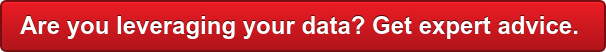# Statistics for Funky Data

The Generalized Linear Model is a huge family of methods widely-used by statisticians. It is typically abbreviated as GLM but is much more than the standard linear regression and ANOVA covered in basic stats courses. That GLM – the General Linear Model – is a subset of this larger family and much more restricted in the kinds of data it can accommodate.

The Generalized Linear Model and its extensions includes binary and multinomial logistic regression, Poisson models for count data, ordinal probit for ordered categorical data, and myriad other advanced models.

Data need not be continuous or normally distributed, and relationships among variables do not have to be straight line. (“Linear” is an ambiguous word in statistics.) Complex interactions – when relationships between variables depend on other variables – can also be modeled.

Data can be very funky these days.

Here are some excellent books on the GLM and advanced variations of it:

• Generalized Linear Models and Extensions (Hardin and Hilbe)
• Regression Modeling Strategies (Harrell)
• Handbook of Quantile Regression (Koenker et al.)
• Generalized Additive Models: An Introduction with R (Wood)
• Vector Generalized Linear and Additive Models (Yee)
• Flexible Regression and Smoothing (Stasinopoulos et al.)
• Nonparametric Econometrics (Li and Racine)
• Applied Nonparametric Econometrics (Henderson and Parmeter)If there are lots of wiggly relationships and interactions among your variables and all you need are predictions – explanation is not critical – neural nets, boosting, bagging and other “machine learning” methods are probably your best choice. They will often get the job done with less headache. Many excellent books on those topics have been published, Data Mining (Whitten et al.), Applied Predictive Modeling (Kuhn and Johnson) and Elements of Statistical Learning (Hastie et al.) being three popular ones.

If explanation – understanding the why – is important, however, then the sorts of regressions covered in the books I listed earlier are probably the way to go. Caveat: they can quickly become complex with more than a few independent variables and the modeler may be faced with a bewildering array of options and decisions, some of them very consequential.

In most data I personally work with, however, interrelationships among variables are weakly linear and differences among groups (e.g., types of consumers) tend to be small. In other words, the challenge is a weak signal, not a complicated one. Nevertheless, explaining whyY behaves the way it does is usually important, and I must be able to make sense of the model.

The approaches outlined in the book by Hardin and Hilbe and the book by Harrell, overall, are the most practical for the sorts of work I do. The books I’ve listed are mainly concerned with supervised learning – when there is a dependent variable. But, like any data, funky data does not need a dependent variable. There are numerous books on unsupervised learning, such as Analyzing Social Networks (Borgatti et al.), Handbook of Cluster Analysis (Hennig et al.) and Correspondence Analysis in Practice (Greenacre). Applied Missing Data Analysis (Enders) is concerned with a special kind of funky data.

I hope you’ve found this brief overview of a very big topic interesting and helpful.

Browse

Article by channel:

Read more articles tagged: Analytics, Featured, Statistics